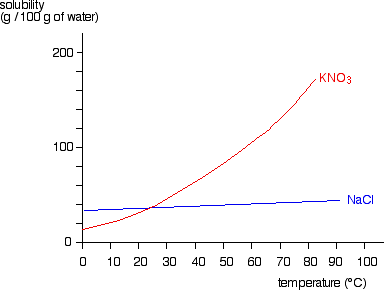The solubility of solids changes as temperature changes. This can be plotted on a solubility curve.The salts shown on this graph are typical: the solubility increases as temperature increases.

For example, the graph above shows that in 100g of water at 50⁰C the maximum mass of potassium nitrate (KNO₃) which will dissolve is 80g.

However, if the temperature were 80⁰C a mass of 160g of potassium nitrate (KNO₃) would dissolve in 100g of water.